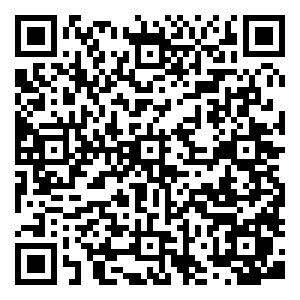## Message Board

Respected readers, authors and reviewers, you can add comments to this page on any questions about the contribution, review,        editing and publication of this journal. We will give you an answer as soon as possible. Thank you for your support!WANG Zhong, PENG Fei, HAN Yuchao, MENG Qingxu, DENG Weiyao. Survey Data Processing Method of Submarine Pressure Resistance Based on Non-uniform Sampling Weighted Least Square Circle Fitting[J]. Geomatics and Information Science of Wuhan University. doi: 10.13203/j.whugis20210675
 Citation: WANG Zhong, PENG Fei, HAN Yuchao, MENG Qingxu, DENG Weiyao. Survey Data Processing Method of Submarine Pressure Resistance Based on Non-uniform Sampling Weighted Least Square Circle Fitting[J]. Geomatics and Information Science of Wuhan University.# Survey Data Processing Method of Submarine Pressure Resistance Based on Non-uniform Sampling Weighted Least Square Circle Fitting

##### doi: 10.13203/j.whugis20210675
Funds:

The National Natural Science Foundation of China(51609253).

• Received Date: 2021-12-02• Objectives: In order to withstand the deep water pressure, the cylindrical structure and the conical structure are often used as the submarine pressure hull. The section roundness of the submarine pressure hull needs to be measured during construction and repair. Due to the limitation of environment conditions and the fusion uses a variety of different survey methods to obtain data, the survey points are often non-uniform. This case would result in the deviation between the results of classical least square circle fitting method and the actual situation, especially to the maximum deflection which used as the judgment condition of roundness. Therefore, it is necessary to adopt a method to eliminate the influence of non-uniform distribution of the survey points. Methods: A non-uniform sampling weighted least squares circle fitting method and its weighting rules were proposed based on the classical Pratt circle fitting method. There are three steps for present method. First, the survey points are fitted by the classical Pratt method. Then, the point weight is calculated according to the corresponding center angle. Finally, the survey points with weight are fitted by present method. Results: Numerical experiments on standard ellipse sampling analysis were taken out. The results shown that the maximum deflection was more affected than the fitted center and radius by the non-uniformity of the sampling points. As the roundness evaluation judgment of the submarine pressure hull is based on the maximum deflection, the fitting results of present method is more accurate and reliable than the classical circle fitting method. Conclusions: The proposed method can eliminate the influence of non-uniform sampling on circle fitting better, and has good accuracy and engineering practical value.
•## Survey Data Processing Method of Submarine Pressure Resistance Based on Non-uniform Sampling Weighted Least Square Circle Fitting

##### doi: 10.13203/j.whugis20210675
###### 1 College of Naval Architecture and Ocean Engineering, Naval University of Engineering, Wuhan 430033, China
Funds:

The National Natural Science Foundation of China(51609253).

Abstract: Objectives: In order to withstand the deep water pressure, the cylindrical structure and the conical structure are often used as the submarine pressure hull. The section roundness of the submarine pressure hull needs to be measured during construction and repair. Due to the limitation of environment conditions and the fusion uses a variety of different survey methods to obtain data, the survey points are often non-uniform. This case would result in the deviation between the results of classical least square circle fitting method and the actual situation, especially to the maximum deflection which used as the judgment condition of roundness. Therefore, it is necessary to adopt a method to eliminate the influence of non-uniform distribution of the survey points. Methods: A non-uniform sampling weighted least squares circle fitting method and its weighting rules were proposed based on the classical Pratt circle fitting method. There are three steps for present method. First, the survey points are fitted by the classical Pratt method. Then, the point weight is calculated according to the corresponding center angle. Finally, the survey points with weight are fitted by present method. Results: Numerical experiments on standard ellipse sampling analysis were taken out. The results shown that the maximum deflection was more affected than the fitted center and radius by the non-uniformity of the sampling points. As the roundness evaluation judgment of the submarine pressure hull is based on the maximum deflection, the fitting results of present method is more accurate and reliable than the classical circle fitting method. Conclusions: The proposed method can eliminate the influence of non-uniform sampling on circle fitting better, and has good accuracy and engineering practical value.

WANG Zhong, PENG Fei, HAN Yuchao, MENG Qingxu, DENG Weiyao. Survey Data Processing Method of Submarine Pressure Resistance Based on Non-uniform Sampling Weighted Least Square Circle Fitting[J]. Geomatics and Information Science of Wuhan University. doi: 10.13203/j.whugis20210675
 Citation: WANG Zhong, PENG Fei, HAN Yuchao, MENG Qingxu, DENG Weiyao. Survey Data Processing Method of Submarine Pressure Resistance Based on Non-uniform Sampling Weighted Least Square Circle Fitting[J]. Geomatics and Information Science of Wuhan University.Reference (5)
PDF viewfollowshare

Top

### CatalogQrcode/DownLoad:  Full-Size Img  PowerPoint